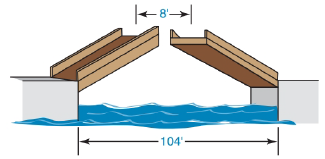Chapter 5.4, Problem 19E### Elementary Geometry for College St...

6th Edition
Daniel C. Alexander + 1 other
ISBN: 9781285195698

#### Solutions

Chapter
Section### Elementary Geometry for College St...

6th Edition
Daniel C. Alexander + 1 other
ISBN: 9781285195698
Textbook Problem
133 views

# A drawbridge that is 104 ft in length is raised at its midpoint so that the uppermost points are 8 ft apart. How far has each of the midsections been raised?To determine

To Find:

Length of the midsections been raised.

Explanation

Given A drawbridge that is 104 ft in length is raised at its midpoint so that the uppermost points are 8 ft apart.

The figure can be redrawn as,

From the given data,

AB = 104 ft.

CD = EF = 8 ft.

And from the redrawn figure,

AE = FB

AC=12(AB)    =12(104)    =52

And from the figure,

AB = AE+EF+FB

104 = AE+8+AE

104 = 2AE+8

2AE = 96

AE = 962

AE = 48

### Still sussing out bartleby?

Check out a sample textbook solution.

See a sample solution

#### The Solution to Your Study Problems

Bartleby provides explanations to thousands of textbook problems written by our experts, many with advanced degrees!

Get Started

#### Evaluate the limit, if it exists. limx4x2+95x+4

Single Variable Calculus: Early Transcendentals, Volume I

#### Let f(x)={2x+1if1x0x2+2if0x2 Find (a) f(1), (b) f(0), and (c) f(32).

Applied Calculus for the Managerial, Life, and Social Sciences: A Brief Approach

#### In Exercise 11-14, factor the expression. y27y+494

Calculus: An Applied Approach (MindTap Course List)

#### Calculate y'. 35. y = cot(3x2 + 5)

Single Variable Calculus: Early Transcendentals

#### Explain the difference between passive and active deception.

Research Methods for the Behavioral Sciences (MindTap Course List)